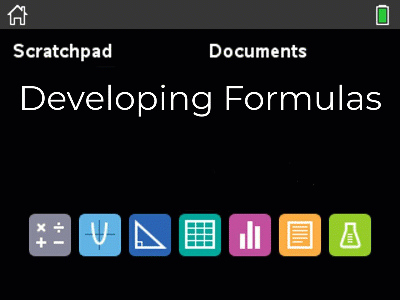# Activities

•• ##### AuthorAust Senior

50 Minutes

• ##### Device
•TI-Nspire™ CX

TI-Nspire™

5.0

## Induction Part 1 - Whole Numbers#### Activity Overview

Part 1 of 3:  Induction for whole numbers requires students to establish a formula for the sum of consecutive whole numbers. Students establish the formula using a variety of techniques and are then required to prove their formula holds true for all whole numbers. (Mathematical Induction). Variations on this summation and formula are also required to be proved by the process of Mathematical Induction.

#### Objectives

Part 1 of 3: Induction - students build equations visually, graphically and numerically and then prove their formula to be true for all whole number by mathematical induction. Additional questions are provided for students to practice the process of mathematical induction.

#### Vocabulary

• Induction
• Sum
• Cumulative sum